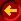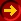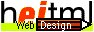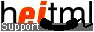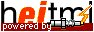# heitml Programs

You can write heitml programs or use heitml features everywhere on a page. heitml is integrated in HTML so there is no special markup necessary to mark heitml tags.

Example:

 input: ``` 1*1=1 , *= ``` resulting output: ``` 1*1=1 , 2*2= 4 , 3*3= 9 , 4*4= 16 , 5*5= 25 , 6*6= 36 , 7*7= 49 , 8*8= 64 , 9*9= 81 , 10*10= 100 , 11*11= 121 , 12*12= 144 , 13*13= 169 , 14*14= 196 , 15*15= 225 , 16*16= 256 , 17*17= 289 , 18*18= 324 , 19*19= 361 ```

When working with RADpage you can enter the example above inside the content box of any component for example of a paragraph component. Note that HTML-tags, like <b> and heitml tags like <?> are mixed.

When using RADpage it is sometimes useful to write programs into the heitmlprog environment. It does actually nothing except showing handles around the program and so simplifying component editing.

## References

Component:heitmlprogDetails
The component is used to encapsulate a program fragment. The component does nothing at page generation time, but the items inside are grouped together and can be handled as a whole in the component editor.

The CDATA construct is required inside to be XML compatible, enter your program inside the square brackets.

This page was dynamically generated by the web application development tool RADpage of H.E.I. See "Was ist AJAX? " (in German). In Germany H.E.I. provides Webdesign in Mannheim and Web Programming (Programmierung).
© 1996-2021 H.E.I. All Rights Reserved.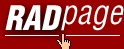Homepage Intro/Features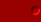Component Guide Application Generic Programmable heitml and HTML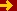heitml Programs Dynamic Properties Control Structures Action Methods FormsProgramming User Guide Services Privacy DatenschutzContact / Impressum